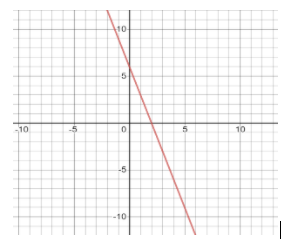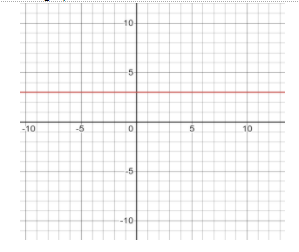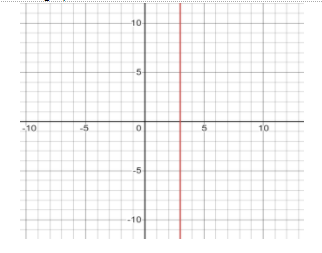Fill in the blanks: the graph of line x +2 = 0, is parallel to …………… axis.Verified
147.6k+ views
Hint: Now, the general from of a linear equation in two variables is given as $ax + by + c = 0$ , with ‘x’ and ‘y’ as variables and ‘a’ and ‘b’ as their coefficients and ‘c’ as the constant. Such that, if $y = 0$ and $x = a$ then the graph of the line will be parallel to the y-axis. On the other hand, if$x = 0$, and $y = b$, then the graph of the line will be parallel to the x-axis.

Now, the standard form of a linear equation in two variables is given as $ax + by + c = 0$, where x and y are the variables and a and b are the coefficients of x and y respectively and c is a constant. Now the graph of the standard form will be a simple line like:Now, in the standard form if we put x = 0, then the equation will change to:
$a(0) + by + c = 0 \\ \Rightarrow 0 + by + c = 0 \\ \Rightarrow by + c = 0 \\ \Rightarrow by = - c \\ \Rightarrow y = - \dfrac{c}{b} \\$
That is the line will be parallel to the x-axis, equidistant from the x-axis at a perpendicular distance of $y = - \dfrac{c}{b}$ , throughout.
The graph for the same will look like:Now, if we put y = 0, then the equation will change to:
$ax + b(0) + c = 0 \\ \Rightarrow ax + 0 + c = 0 \\ \Rightarrow ax + c = 0 \\ \Rightarrow ax = - c \\ \Rightarrow x = - \dfrac{c}{a} \\$
That is the line will be parallel to the y-axis, equidistant from the y-axis at a perpendicular distance of $x = - \dfrac{c}{a}$ , throughout.
The graph for the same will look like:Hence, the graph of line$x + 2 = 0$ is parallel to ……y……… axis.

Note: When we are putting the value of either x or y as 0, in the standard form of linear equation in two variables, the signs have to be written very carefully. Since, if the sign is wrong, then the axis whose perpendicular distance is being considered will change, however the line will still remain parallel and the distance will remain the same but on the other axis. For example, if instead of x + 2 = 0 which gives us x = -2, instead of taking the value of x as -2 if by mistake we take it as x = 2, then the line will still be parallel to the y-axis only, but always at a perpendicular distance of 2 units away from the y-axis, on the positive x-axis.